gas equations equations graph equations find equations differential equations innovative equations solve chemcial equations balancing chemical equations system of differential... ordinary differential... simultaneous linear equations systems of partial...
Дом и Обучение :: Математика
Аудио :: Всё-в-одном
Дом и Обучение :: Наука## Easy Equation Solver

Easy Equation Solver is a simple and easy to use program for solving equations. The program can solve: Quadratic Equations, Cubic Equations, 2 Equations with 2 Unknowns, 3 Equations with 3 Unknowns, Determinants 2x2 and Determinants 3x3.

Ключевые слова:
equation solver, quadratic, cubic, unknowns, equations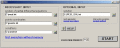## PEEI

The program PEEI 4.0.0.0 calculates numerical solutions of systems of partial differential equations that have number of equations equal or greater of the number of unknown functions.## Chemical Equation Expert

Chemical Equation Expert is an integrated tool for chemistry professionals and students. You'll find complicated work such as balancing chemical equations and related calculations so easy and even enjoyable!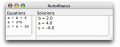## AutoAbacus

AutoAbacus is a powerful equation solving library that finds solutions to equation sets with a snap. A set of equations can be passed in as text, while AutoAbacus attempts to find a solution that satisfies all constraints.

Ключевые слова:
autoabacus, java, business rules, rules engine, math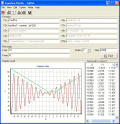## Equation Plotter - EqPlot

Graph plotter program plots 2D graphs from complex equations. The application comprises algebraic, trigonometric, hyperbolic and transcendental functions. EqPlot can be used to verify the results of nonlinear regression analysis program.

Ключевые слова:
equation, graphing, graph, plotter, eqplot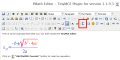## FMath Editor - TinyMCE Plugin

fMath Editor -TinyMCE plugin is the best math editor to create and edit equations on web. It has more than 20000 symbols to display and a rich interface. The formula can edited by interface or in MathML or in LaTeX.

Ключевые слова:
TinyMCE, fMath Editor, mathematics, equations, formula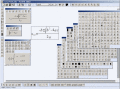## FMath Editor - CKEditor Plugin

fMath Editor -CKEditor plugin is the best math editor to create and edit equations on web. It has more than 20000 symbols to display and a rich interface. The formula can edited by interface or in MathML or in LaTeX.

Ключевые слова:
CKEditor, fMath Editor, mathematics, equations, formula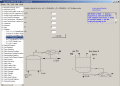## EquationsPro

EquationsPro s a chemical engineering,mathematical program. solves 400+ chemical/electrical/mechcanical engineering, physics, and mathematical equations. Contains 200+ unit conversions, Solve and plot graphs using the Zgraphs program. & more.

Ключевые слова:
engineering tools, chemical engineering, chemistry, science, unit conversions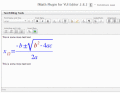## FMath Editor - YUI Editor Plugin

fMath Editor -YUI Editor plugin is the best math editor to create and edit equations on web. It has more than 20000 symbols to display and a rich interface. The formula can edited by interface or in MathML or in LaTeX.

Ключевые слова:
YUI Editor, Yahoo, fMath Editor, mathematics, equations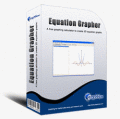## Equation Grapher

Equation grapher is a free graphing calculator to graph equations. Equation Grapher is a free graphing calculator to graph equations. Equation Grapher is a free graphing calculator to graph equations.

Ключевые слова:
equation grapher, free graphing calculator, graph equation, graph equations

Страница: 1 | 2 | 3 | 4 | 5
 Категории: Главная Аудио Бизнес Рабочий Стол Инструменты Разработчика Игры Дом и Обучение Интернет Мультимедия Утилиты Видео Вёб Разработка Свежий софт: Stockalyze TS School Hide.me VPN for Windows Account Hacker Email Hacker